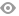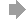﻿ Page 7 - Mathematics Literacy Grade 11
Basic HTML VersionView Full Version
Page 7 - Mathematics Literacy Grade 11
P. 7

``````Unit 2: Constant difference relationship

A constant difference relationship may be described as a relationship between two
variables such that a change in one means a uniform change in the other. Also a
decrease in one variable will cause a decrease in the other.

Example 2

A metered taxi  charges R5,  00 per kilometer  travelled. We could create a
table to look at the relationship between kilometers and cost:

Number of                     1  2                3               4              5
kilometers (  )
Amount in rands               5  10               15              20             25
(R)

We can see that there is a constant difference between the variables in the above
table. We could create an equation to represent the above relationship: R= 5  . If
Peter travelled in the taxi for 12 kilometers we would work it out by multiplying the
number of kilometers by R5, 00 so our answer will be R60.

If we drew this relationship on a graph it would look like:

30
25
20
Rands   15
10
5
0
0      1      2      3      4      5      6
Kilometers

6 | P a g e                             I v y   A c a d e m y``````2   3   4   5   6   7   8   9   10   11   12